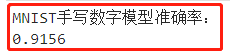用 Notebook 实现手写数字识别

数据集介绍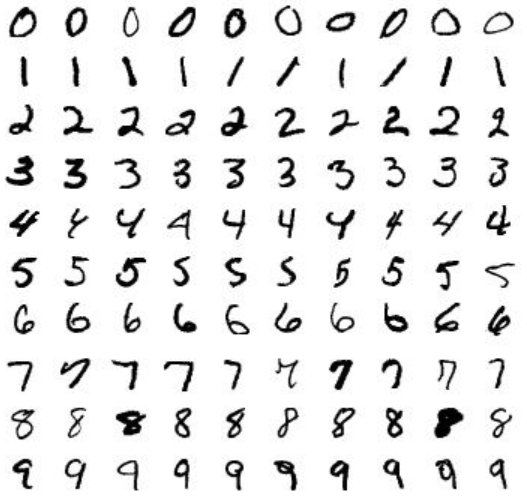整体流程

1. 新建 Notebook 容器
2. 在 Notebook 中，创建 MNIST 手写数字识别项目
• 创建 Python 文件
• 从 MNIST 官网下载数据集并上传到项目文件夹中
3. 利用 TensorFlow 实现手写数字识别：
• 安装并导入所需依赖包
• 加载 MNIST 数据集
• 数据集可视化展示
• 构建手写数字识别模型
• 模型评估输出模型准确率

详细流程

新建 Notebook 容器

1. 登录智能钛机器学习平台控制台后，单击顶层菜单栏的【Notebook】，页面跳转至 Notebook 容器列表页面。单击【新增】，配置容器参数：
• 容器名称：MNIST 手写数字识别
• 框架选择：tensorflow/1.12
• 资源选择：后付费/CPU/2核4G内存
• 存储桶：此处请选择您自己在 COS 上的存储桶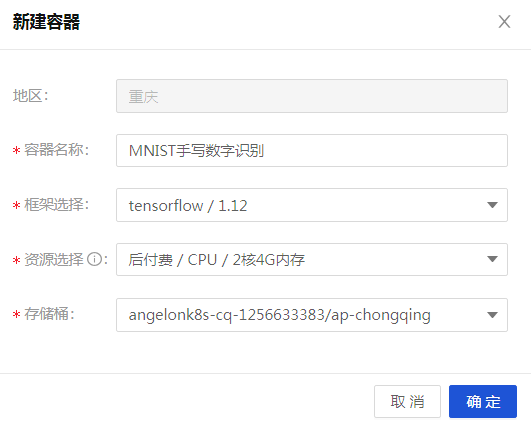1. 待 Notebook 容器创建完成后状态为：运行中，单击【打开】进入 Notebook 操作页面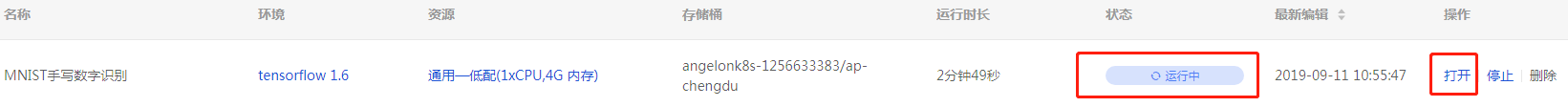创建 MNIST 手写数字识别项目

1. 在 Notebook 操作页面，选择【Python3】，进入项目后，将项目重命名为：MNIST.ipynb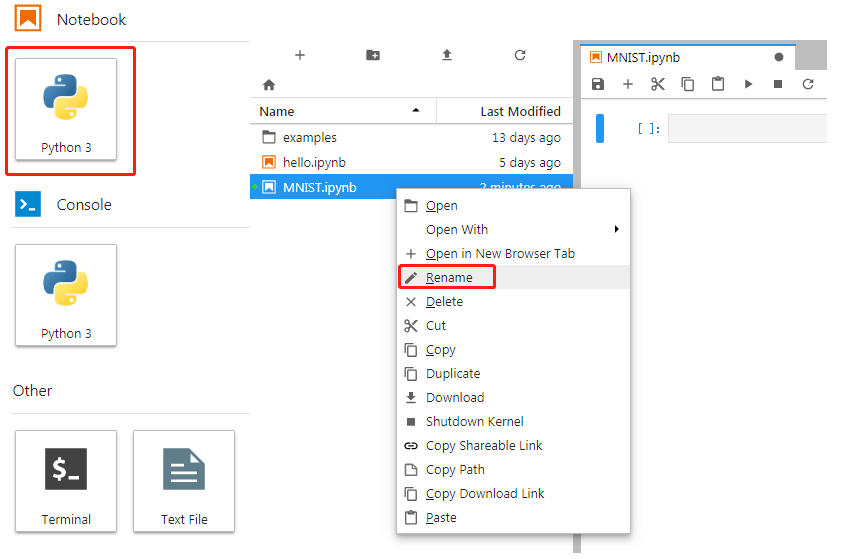2. 在 MNIST.ipynb 的同级目录处，新建文件夹【MNIST_data】，并将 MNIST 官网 提供的四个数据集下载后上传至该文件夹中：
• train-images-idx3-ubyte.gz
• train-labels-idx1-ubyte.gz
• t10k-images-idx3-ubyte.gz
• t10k-labels-idx1-ubyte.gz利用 TensorFlow 实现 MNIST 手写数字识别

1. 在 Notebook 中安装并导入所需依赖包

<!--您可直接复制以下所有代码块到 Notebook 中运行-->
! pip install --user --upgrade pip
! pip install --user tensorflow

import matplotlib.pyplot as plt
import tensorflow as tf
from tensorflow.examples.tutorials.mnist import input_data
from tensorflow.examples.tutorials.mnist import input_data
2. 加载 MNIST 数据集

mnist = input_data.read_data_sets("MNIST_data/", one_hot=True)
3. 可视化查看数据内容
选择数据集中的一个示例打印出来，查看数据内容。

说明：

此处打印的数据每次都是随机的，可能内容不一样。

im = mnist.train.images.reshape(28, 28)
plt.imshow(im, cmap='Greys')
plt.show()
print(mnist.train.labels)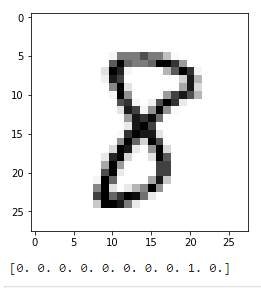1. 搭建手写数字识别模型

# 模型构建
sess = tf.InteractiveSession()
x = tf.placeholder(tf.float32, [None, 784])
W = tf.Variable(tf.zeros([784,10]))
b = tf.Variable(tf.zeros())

y = tf.nn.softmax(tf.matmul(x,W) + b)

y_ = tf.placeholder(tf.float32, [None,10])
cross_entropy = tf.reduce_mean(-tf.reduce_sum(y_ * tf.log(y),reduction_indices=))

# 模型训练
tf.global_variables_initializer().run()

for i in range(1000):
batch_xs, batch_ys = mnist.train.next_batch(100)
train_step.run({x: batch_xs, y_: batch_ys})
2. 模型评估

correct_prediction = tf.equal(tf.argmax(y,1), tf.argmax(y_,1))
accuracy = tf.reduce_mean(tf.cast(correct_prediction, tf.float32))

print('MNIST手写数字模型准确率：')
print(accuracy.eval({x: mnist.test.images, y_: mnist.test.labels}))